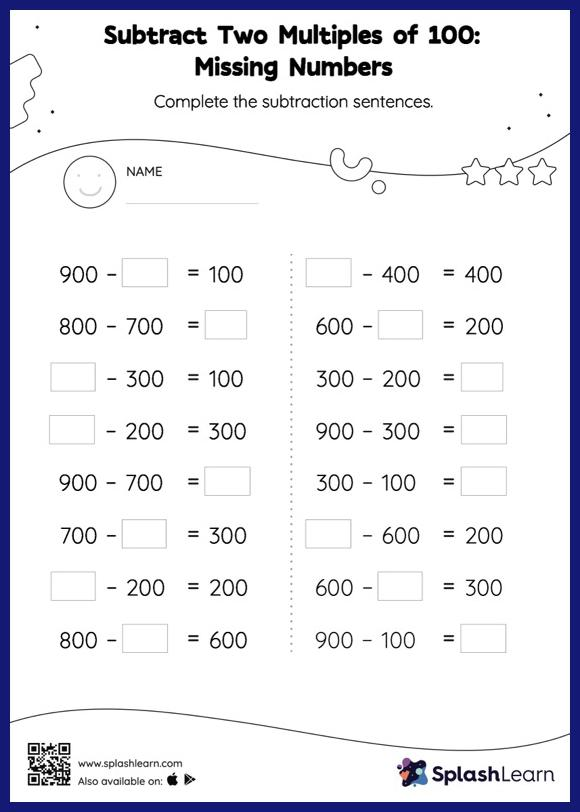# Subtract Two Multiples of 100: Missing Numbers Worksheet

Home > Subtract Two Multiples of 100: Missing NumbersStudents use the count-back method or the relationship between addition and subtraction to find the missing number if it is a minuend or subtrahend. Also, they subtract the numbers to get the difference if the missing number is the difference. There is a lot of practice with this concept in the worksheet subtract two multiples of 100. As the worksheet uses a horizontal format, it allows for more creativity when it comes to how the student solves the problem. In contrast, in the vertical format, students usually employ the standard method to solve.